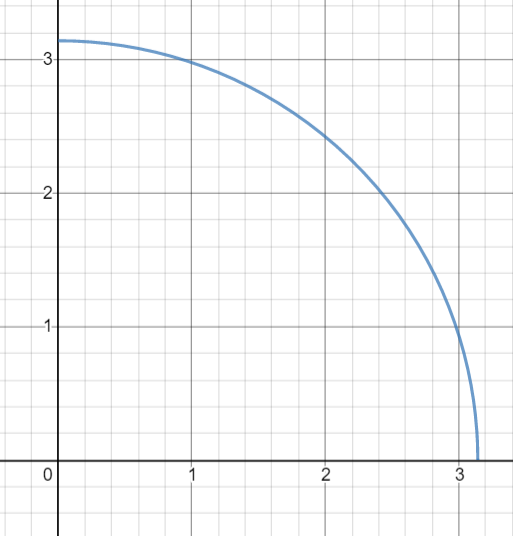# May the Potential Energy be with you!A chain of mass $\underline{\red{5 \text{ kg}}}$ of uniform density is kept above the surface of the Earth in the form of a quarter circle of radius $\underline{\red{{\pi \text{ m}}}}$ as shown in the figure. Find the total potential energy of the chain at this position, in joules, upto 2 decimal places.

#### $\underline{\red{\text{Details and assumptions.}}}$

• There is no air resistance.

• Take $\purple{\underline{\text{Acceleration due to gravity = 10 ms}^{-2}}}$

• Potential energy at the surface of the Earth $=0$

• This problem is not original, but the graph is plotted and edited by me.

• I will be very glad and grateful if you post a solution.

×